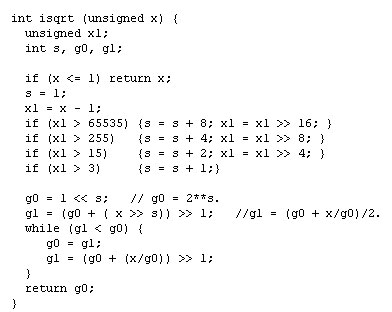Search

Useful Lists

Partners

Online Manuals

Hacker's Delight
By Susan McVey - 2004-03-26 Page:  1 2 3

## Code for Simple to Complex Mathematical Operations

In the course of everyday software engineering, most of us just link our programs with a standard math library and take it for granted that the machine knows how to perform multiplication, division, and other operations on unsigned and signed integers, long integers, floating-point numbers, and so on. This book goes into greater detail, explaining exactly how to do each of these calculations.

After disposing of basic arithmetic operations, Warren turns his attention to more complex math problems -- calculating square roots, for example. His discussion of the subject is both complex and simple. First, he explains Newton's method of computing square roots through a page full of equations that require some effort to follow -- but then he gives an implementation that requires fewer than twenty lines of C code. This is followed by another method that is longer and more cryptic but executes faster, by using a binary search algorithm. Whether you are interested in the equations or merely need the C code to do your job, Warren's solutions are efficient and elegant. Here is his code for computing an integer square root using Newton's method:The book also offers similar solutions for computing cube roots, exponents, and logarithms.

View Hacker's Delight Discussion

Page:  1 2 3 Next Page: Pursuit of Fascinating Problems